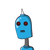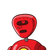# In a business, the ratio of investments of A, B and C is 5:6: 7. The ratio of profitsat the end of one year is 5 : 9 :

In a business, the ratio of investments of A, B and C is 5:6: 7. The ratio of profits
at the end of one year is 5 : 9 : 14. Find the
ratio of the respective periods of their
investments.

### 2 thoughts on “<br />In a business, the ratio of investments of A, B and C is 5:6: 7. The ratio of profits<br />at the end of one year is 5 : 9 :”

1.2:3:4

Clue:

Profits are directly proportional to time and investment.

2.The ratio of Profit is, 5 : 9 : 14

So, P1 : P2 : P3 = 5 : 9 : 14

Also, ratio of investments is 5 : 6 : 7

So, X1 : X2 : X3 = 5 : 6 : 7

Now, we have to find the ratio of their period of investments.

So, T1 : T2 : T3 = P1/X1 : P2/X2 : P3/X3

So, T1 : T2 : T3 = 5/5 : 9/6 : 14/7

So, T1 : T2 : T3 = 1 : 1.5 : 2

Or T1 : T2 : T3 = 2 : 3 : 4

So, the ratio of period of investments is 2 : 3 : 4

hope it’s helps you